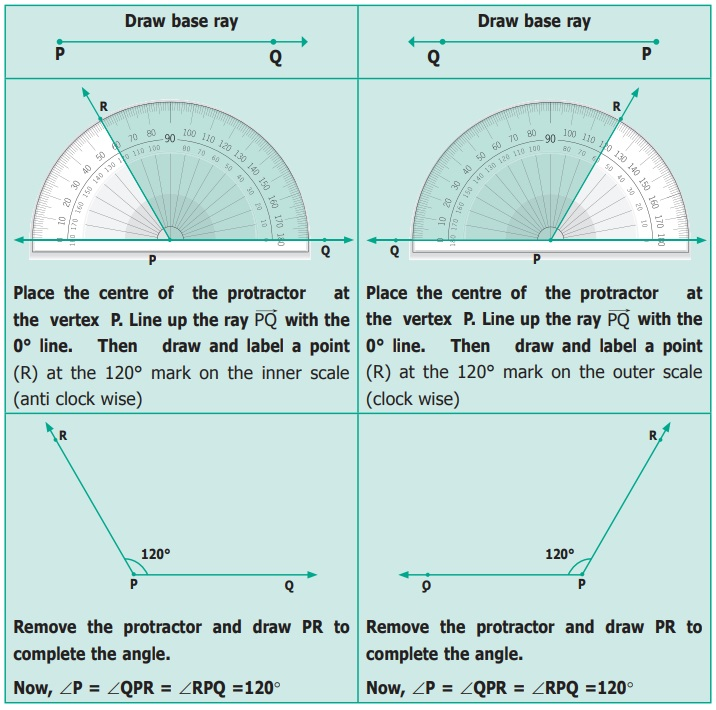Home | | Maths 6th Std | Angle measurement using Protractor

# Angle measurement using Protractor

How do we measure an angle? Using a PROTRACTOR we can measure an angle.

Angle measurement using Protractor

How do we measure an angle? Using a PROTRACTOR we can measure an angle.A protractor has one centre and a base line. It has two scales namely, inner scale from 0° to 180° in one direction and outer scale from 0° to 180° in the opposite direction. Why does the protractor stop with 180°? We can rotate the protractor and measure, so 180° is enough.

Steps To Measure an angle

Step 1 Place the centre of the protractor on the vertex of the angle and line up the base line with 0°.

Step 2 Read the measure where the other ray crosses the protractor.

1. Using Protractor to draw Right Angle (90º)

Example

Use a Protractor to draw an angle 90º.2. Using Protractor to draw an Acute Angle

Example

Use a Protractor to draw an angle 45°.3. Using Protractor to draw an Obtuse Angle

Example

Use a protractor to draw an obtuse angle 120°.Reflex angles are bigger than 180°. Always subtract the given angle from 360° to get the reflex angle.Activity

Fix a line segmentand let’s have another line segment, go on rotating AC to the left.At some point,overlapand then we are back to the same as before. So angle, keep on increasing and after some point, return to 0º.

Is this familiar? Yes, you can see this in a clock!

Try this

Adjust the hands of the clock for the following time, note the angle made between the hour hand and the minute hand and write the type of angle.Tags : Geometry | Term 1 Chapter 4 | 6th Maths , 6th Maths : Term 1 Unit 4 : Geometry
Study Material, Lecturing Notes, Assignment, Reference, Wiki description explanation, brief detail
6th Maths : Term 1 Unit 4 : Geometry : Angle measurement using Protractor | Geometry | Term 1 Chapter 4 | 6th Maths